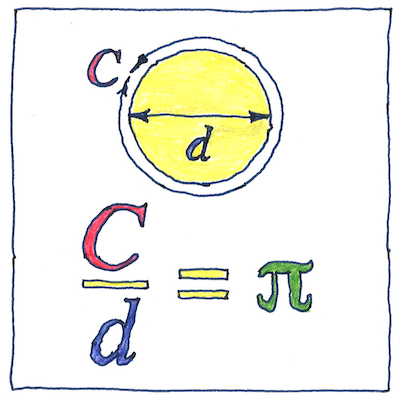# 1768

## The book of science

Tom Sharp

 Johann Heinrich Lambert, Ferdinand von Lindemann mathematics

## Pi

• The history of π
• (the ratio
• of the circumference to the diameter of a circle)
• is old.
• The Egyptians knew of it;
• the Babylonians knew of it;
• the Greek mathematician Archimedes
• used a polygon of ninety-six sides
• to calculate it;
• the Chinese mathematician Liu Hui knew of it
• and used polygons of one hundred ninety-two sides
• and three thousand seventy-two sides,
• calculating their areas rather than perimeters.
• Over the centuries, new methods for calculating π
• have resulted in increasing accuracy—
• but all decimal representations are approximations
• of a number that is
• never ending
• and never repeating.

## Proofs

• In 1768, Johann Heinrich Lambert
• proved that π is irrational
• it cannot be expressed as a ratio of whole numbers
• and cannot have a repeating pattern.
• In 1882, Ferdinand von Lindemann
• proved that π is transcendental
• it cannot be a root of a polynomial equation
• with rational coefficients.
• One hopes, by now, inquiring minds have turned
• to problems that can be solved.

## Squaring the circle

• The eye encompasses the obvious only
• and often is mistaken.
• Then the mind follows, convinced it should believe
• what it has seen.
• It doesn’t matter how hard we try
• to turn a base metal into gold
• to build a perpetual motion machine
• or to square the circle,
• human effort cannot controvert
• the intricate and subtle
• nature of our physical universe.
• Seldom, if ever, do we exactly
• hit the mark.

To “square the circle” is to find the length of the sides of a square with the same area as a circle of a given radius. The area of a circle of radius ``` r ``` is ``` πr2 ``` , so success depends on the nature of π. The proofs of Lambert and Lindemann mean that we know that it is impossible for anyone to construct on a flat surface a square with the same area as a given circle using a compass and ruler.

The expression “squaring the circle” means trying to do the impossible.

Archimedes used the “method of exhaustion” to approximate the value of π. The work of both Archimedes and Liu Hui prefigured calculus; Archimedes wrote of infinitesimals and Liu Hui described the use of the limit.